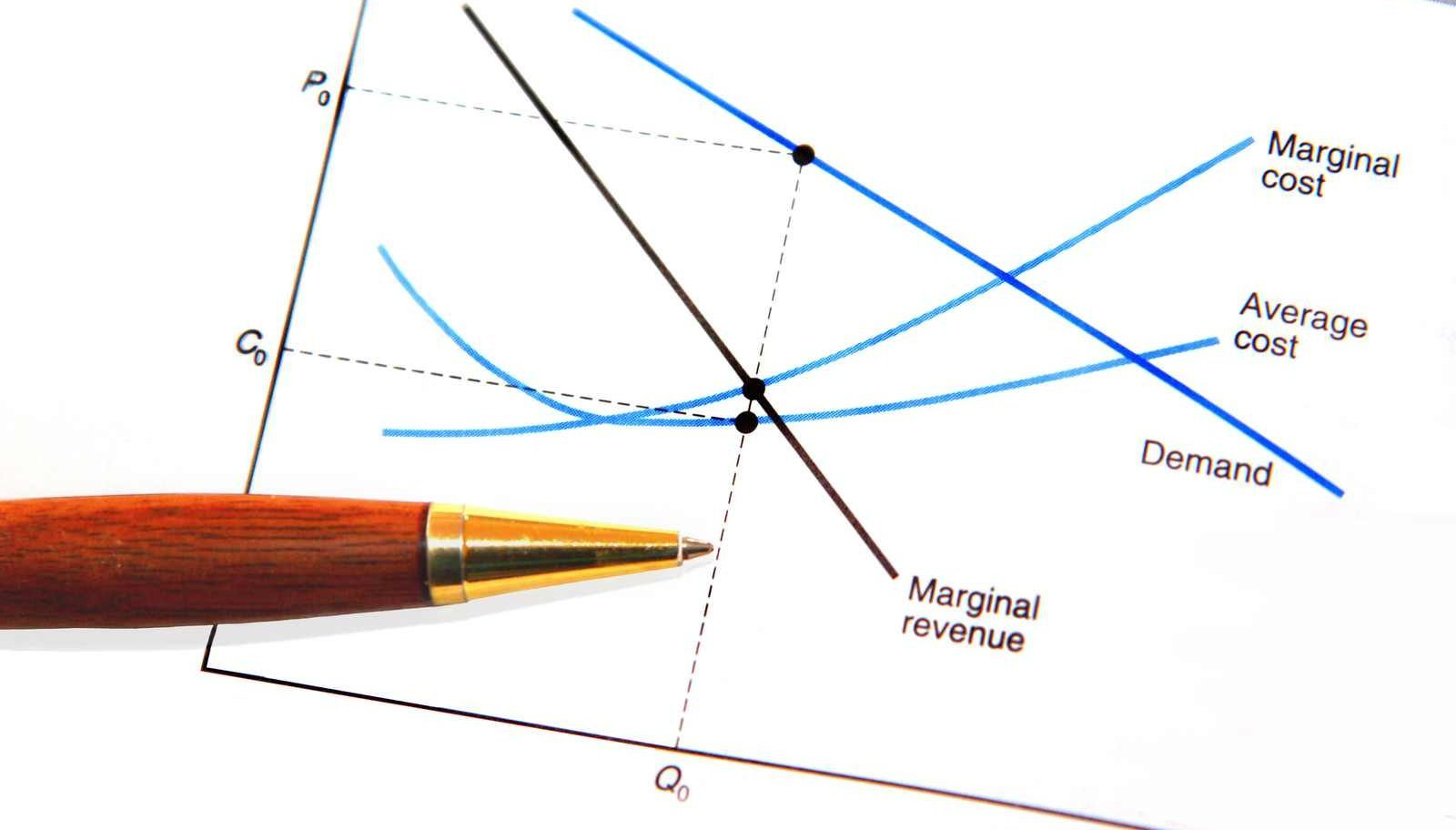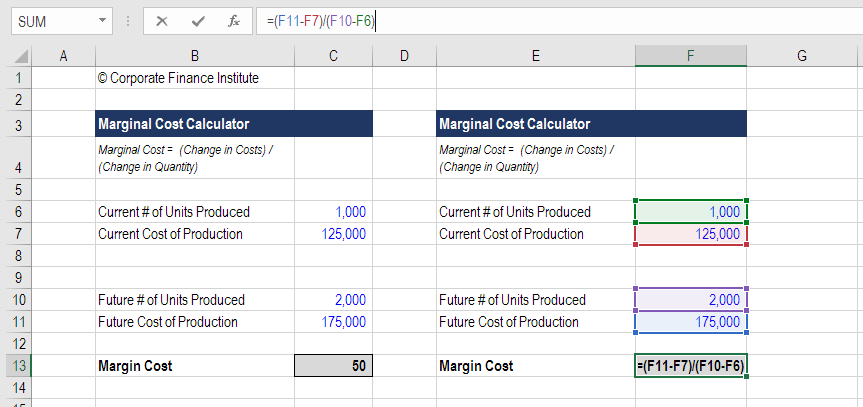# Marginal Cost Formula

The cost of producing one more unit

## What is Marginal Cost?

Marginal cost represents the incremental costs incurred when producing additional units of a good or service. It is calculated by taking the total change in the cost of producing more goods and dividing that by the change in the number of goods produced. The usual variable costs included in the calculation are labor and materials, plus the estimated increases in fixed costs (if any), such as administration, overhead, and selling expenses. The marginal cost formula can be used in financial modeling to optimize the generation of cash flow.

Below we break down the various components of the marginal cost formula.Image: CFI’s Budgeting & Forecasting Course.

### What is the Formula for Marginal Cost?

The Marginal Cost Formula is:

#### 1. What is “Change in Costs”?

At each level of production and during each time period, costs of production may increase or decrease, especially when the need arises to produce more or less volume of output. If manufacturing additional units requires hiring one or two additional workers and increases the purchase cost of raw materials, then a change in the overall production cost will result. To determine the change in costs, simply deduct the production costs incurred during the first output run from the production costs in the next batch when output has increased.

#### 2. What is “Change in Quantity”?

It’s inevitable that the volume of output will increase or decrease with varying levels of production. The quantities involved are usually significant enough to evaluate changes in cost. An increase or decrease in the volume of goods produced translates to costs of goods manufactured (COGM). To determine the changes in quantity, the number of goods made in the first production run is deducted from the volume of output made in the following production run.

How do you calculate the marginal cost? Download CFI’s free Marginal Cost Calculator. If you want to calculate the additional cost of producing more units, simply enter your numbers into our Excel-based calculator and you’ll immediately have the answer.

Begin by entering the starting number of units produced and the total cost, then enter the future number of units produced and their total cost.  The output of that equation is the marginal cost. Below is a screenshot of the calculator.### Marginal Cost Calculator

Enter your email and we’ll send you this exclusive marginal cost formula calculator in Excel for yours to keep.

### An Example of the Marginal Cost Formula

Johnson Tires, a public company, consistently manufactures 10,000 units of truck tires each year, incurring production costs of \$5 million. However, one year finds the market demand for tires significantly higher, requiring the additional production of units, which prompts management to purchase more raw materials and spare parts, as well as to hire more manpower. This demand results in overall production costs of \$7.5 million to produce 15,000 units in that year.  As a financial analyst, you determine that the marginal cost for each additional unit produced is \$500 (\$2,500,000 / 5,000).

### How Important is Marginal Cost in Business Operations?

When performing financial analysis, it is important for management to evaluate the price of each good or service being offered to consumers, and marginal cost analysis is one factor to consider.

If the selling price for a product is greater than the marginal cost, then earnings will still be greater than the added cost – a valid reason to continue production. If, however, the price tag is less than the marginal cost, losses will be incurred and therefore additional production should not be pursued – or perhaps prices should be increased. This is an important piece of analysis to consider for business operations.

### What Jobs Use the Marginal Cost Formula?

Professionals working in a wide range of corporate finance roles calculate the incremental cost of production as part of routine financial analysis.  Accountants working in the valuations group may perform this exercise calculation for a client, while analysts in investment banking may include it as part of the output in their financial model.

### Video Explanation of Marginal Cost

Below is a short video tutorial that explains what marginal cost is, the formula to calculate it, and why it’s important in financial analysis.

Video: CFI’s Financial Analysis Courses.

### Economies of Scale (or Not)

Businesses may experience lower costs of producing more goods if they have what are known as economies of scale.  For a business with economies of scale, producing each additional unit becomes cheaper and the company is incentivized to reach the point where marginal revenue equals marginal cost. An example would be a production factory that has a lot of space capacity and becomes more efficient as more volume is produced. In addition, the business is able to negotiate lower material costs with suppliers at higher volumes, which makes variable costs lower over time.

For some businesses, per unit costs actually rise as more goods or services are produced. These companies are said to have diseconomies of scale.  Imagine a company that has reached its maximum limit of production volume. If it wants to produce more units, the marginal cost would be very high as major investments would be required to expand the factory’s capacity or lease space from another factory at a high cost.

We hope this has been a helpful guide to the marginal cost formula and how to calculate the incremental cost of producing more goods.  For more learning, CFI offers a wide range of courses on financial analysis, as well as accounting, and financial modeling, which includes examples of the marginal cost equation in action.

More learning: Browse many of our FREE Finance courses.

• Operating margin
• EBITDA margin
• Profit margin
• Contribution margin